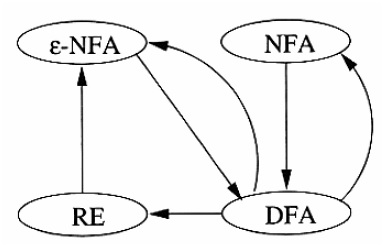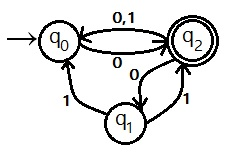Finite automata include two different classes Deterministic Finite Automata (DFA) and the Non deterministic Finite Automata (NFA). These are converted from one to another i.e. Non Deterministic Finite Automata to Deterministic Finite Automata (NFA toDFA) conversion and Deterministic Finite Automata to Non Deterministic Finite Automata (DFA to NFA) conversion. Some more enhancement of NFA machine is NFA with ε moves i.e. (ε-NFA) can be convert into DFA and vice versa. A DFA is directly converted into Regular expression. Below diagram explain about all conversions of Finite Automata conversion.Figure (1) : (NFA→ DFA) and (DFA→ NFA) conversion block diagram

## Non Deterministic Finite Automata to Deterministic Finite Automata (NFA to DFA conversion) :

The goal is to construct a Deterministic Finite Automata (DFA) from given Non-Deterministic Finite Automata (DFA) machine which is much faster in recognition an input string. The main idea behind this conversion to construct a DFA machine in which each state will corresponds to a set of NFA states.

### Worst Case complexity of (NFA→ DFA) conversion:

The worst case complexity of NFA to DFA conversion is O(2N) of sets of states. The DFA can have exponentially many more states than the NFA in rare cases.

## NFA to DFA conversion Algorithm:

Input:
A NFA                         S = States = { s0, s1, …, sN} = SNFA
δ = Move function = MoveNFA
Move’(S, a) → Set of states

Output:
A DFA                         S = States = {?, ?, …, ?} = SDFA
δ = Move function = MoveDFA
Move(s, a) → Single state from SDFA

Main idea:
Each state in SDFA will be a set of states from the NFA SDFA = { {…}, {…} , …, {…} }
(The name of the states in constructed DFA is arbitrary and can be changed later, if desired.)

Method:

Construct a transition table TransactionD. Each DFA state is a set of NFA states. TransactionD simulates in parallel all possible moves N can make on a given string.

Operations to keep track of sets of NFA states:

ε Closure (S):
Set of states reachable from state S via epsilon.

ε Closure (T):
Set of states reachable from any state in set T via epsilon.

move (T, a):
Set of states to which there is an NFA transition from states in T on a symbol a.

### Algorithm:

initially, ε-Closure (S0) in TransactionD.
While unmarked state T in TransactionD
mark T
for each input symbol ‘a’
do u = ε Closure (T, a)
If u is not in TransactionD
TransactionD [T, a] = U

Following algorithm shows a computation of ε -Closure function.
Push all states in T onto stack.
initialize ε-Closure (T) to T
while stack is not empty
do pop top element t
for each state u with ε-edge
t to u
do If u is not in ε-Closure(T)
do add u ε Closure (T)
push u onto stack

### Remarkable Steps to convert NFA→ DFA:

1. Construct the transaction table of given NFA machine.
2. Scan the next states column in the transaction table from initial state to final state.
3. If any of the next state consists more than one state on the single input alphabet. Then merge them and make it new state. Place this new constructed state in DFA transaction table as present state.
4. The next state of this new constructed state on input alphabet will be the summation of each next state which parts in the NFA transaction table.
5. Repeat step 2 to step 4 until all the states in NFA transaction table will be scanned completely.
6. The finial transaction table must have single next state at single input alphabet.

Example:Figure (1): NFA

Construct the equivalent DFA of the NFA given in figure (1).
Step 1: Transaction Table of NFA from Figure (1):

 Present State Next State 0 1 →q0 {q2} Φ q1 Φ {q0, q2} q2* {q0, q1} {q0}

Step 2: Transaction Table of DFA:

 Present State Next State 0 1 →{q0} {q2} Φ {q2} {q0, q1} {q0} {q0, q1} {q2} {q0, q2} {q0, q2} {q0, q1, q2} {q0} {q0, q1, q2} {q0, q1, q2} {q0}
1.Praveen Saini
2.lucy